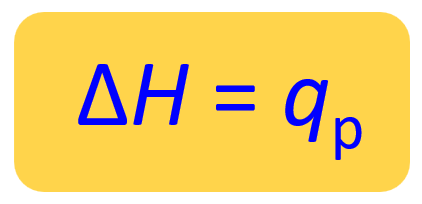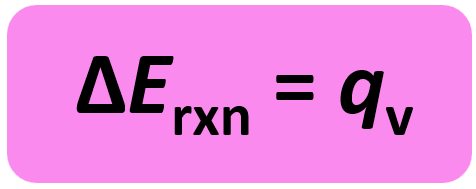## General Chemistry

What is enthalpy in simple words?

Answering this question is the objective of today’s post.

Remember, in the previous post, we talked about internal energy and how it can change by either heat or work.Rearranging this equation for q, we get:

q = ΔE – w, and because w = -PΔV, we can write that:

q = ΔE + PΔV

For a reaction carried out at constant pressure in an open container, q is simply replaced with a special symbol ΔH which is the enthalpy change of the reaction:

ΔH = ΔE + PΔV

Therefore, for a reaction at constant pressure, the enthalpy change is equal to the heat of the reaction (ΔH = qp).We can show this by plugging in the expression ΔE = q +w in the enthalpy change equation:The enthalpy (H) itself would be equal to:

H = E + PV

where E is the internal energy of the system, and P and V are the pressure and volume of the system, respectively.

So, the enthalpy (H) of a system is the sum of its internal energy and the product of its pressure and volume. And because E, P, and V are all state functions, enthalpy is also a state function which only depend on the initial and final states and not how it was achieved.

For example, altitude is a state function and wouldn’t matter how two hikers meet at a 500 ft altitude. One may be on his way to the top, and the other going back having behind a longer walk distance:For chemical reactions, we are more interested in the enthalpy change, which, because is a state function, is equal to the difference of the sums of enthalpies of product and reactants:

ΔH  = HproductsHreactants

However, measuring the absolute enthalpy of a compound is impossible, and therefore, the term “standard enthalpy of formation” is used which is the enthalpy change when one mole of a substance is formed from free elements in their standard/free form.

Just like the heat and ΔE, the sign for the enthalpy change is positive for endothermic reactions and negative for exothermic reactions:Let’s summarize what we learned about enthalpy:

• Enthalpy is a state function.
• Under constant pressure, the ΔHof a reaction is the amount of heat (q) absorbed or evolved in the reaction.
• An endothermic reaction absorbs heat from the surroundings and has a positive ΔH
• An exothermic reaction releases heat to the surroundings and has a negative ΔH

At this point, we are done defining enthalpy and the next part is only if you’d like to answer the following question:

# What About Reactions at Constant Volume?

So far, we discussed reactions at constant pressure which are the most common in chemistry. And for these, we saw that the enthalpy change is the heat emitted or absorbed by the reaction.

Another type of reactions is carried out under constant pressure, and for this, we also use the internal energy equation:

ΔE = q + w

w = PΔV, and ΔV is zero since the volume is constant. So, for a constant volume:So, when we measure the heat of a reaction carried out at a constant volume, we obtain the energy change of the reaction. Both ΔH and ΔE represent changes in a state function for the system, and therefore, conceptually ΔH and ΔE are similar.

The ΔE is a measure of all of the energy (heat and work) of the reaction, while ΔH is a measure of only the heat exchanged under conditions of constant pressure.

For reaction associated with little or no volume change, P ΔV, and thus, the work exchange is zero, is zero ΔH and ΔE are nearly identical in value.

For example, the combustion reaction of propane (C3H8) produces 2044 kJ of heat when the reaction is carried out in an open container at constant pressure:

C3H8(g) + 5O2(g) → 3CO2(g) + 4H2O(l) ΔH =2044 kJ

However, when the reaction is carried out in a sealed container such that PV = 0 and work is possible, all the energy is released as heat, and it is a higher number: ΔE = -2046 kJ.

The difference is only 2 kJ which is the expansion work done against the atmosphere as 6 mol of gaseous reactants are converted into 7 mol of gaseous products.

So, to summarize,

Most of the time, ΔErxn and ΔHrxn are approximately equal but pay attention to what the problem asks to determine.

The energy change of reactions carried out in sealed containers is measured by bomb-calorimetry which we will cover in a separate post.

For the enthalpy change of reactions at constant pressure, check the post Constant-Pressure Calorimetry.

Check Also